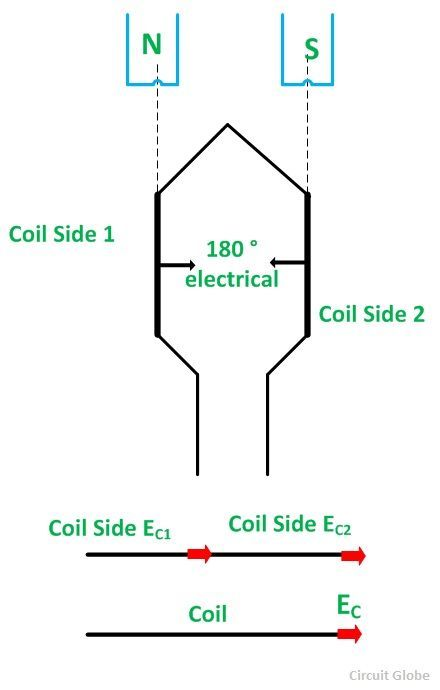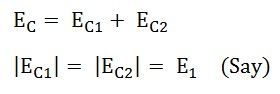# Coil Span Factor

The Coil Span Factor or Pitch Factor KC is defined as the ratio of the voltage generated in the short pitch coil to the voltage generated in the full pitch coil. The distance between the two sides of a coil is called the Coil Span or Coil Pitch Factor. It is also known as Chording Factor.

The angular distance between the central line of one pole to the central line of the next pole is called Pole Pitch. A pole pitch is always 180 electrical degrees, regardless of the number of poles on the machine. A coil having a span equal to 180⁰ electrical is called a full pitch coil as shown in the figure below:A coil having a span less than 180⁰ electrical is called a short pitch coil or fractional pitch coil. It is also called a chorded coil. The short pitch coil factor is shown in the figure below:A stator winding using fractional pitch coil is called a chorded winding. If the span of the coil is reduced by an angle α electrical degrees, the coil span will be (180 – α) electrical degrees.

In case of a full pitch coil, the distance between the two sides of the coil is exactly equal to the pole pitch of 180⁰ electrical. As a result, the voltage in a full pitch coil is such that the voltage of each side of the coil is in phase.

Let EC1 and EC2 be the voltages generated in the coil sides, and EC is the resultant coil voltage.

Then the equation is given as shown below:Since EC1 and EC2 are in phase, the resultant coil voltage EC is equal to their arithmetic sum.

Therefore,If the coil span of a single coil is less than the pole pitch of 180⁰ electrical, the voltages generated on each coil side are not in phase. The resultant coil voltage EC is equal to the phasor sum of EC1 and EC2

If the coil span is reduced by an angle α electrical degrees, the coil span is (180 – α) electrical degrees. The voltage generated EC1 and EC2 in the two coil sides will be out of phase with respect to each other by an angle α electrical degrees. The phasor sum of EC1 and EC2 is EC, which is equal to AC as shown in the phasor diagram above.

The coil span factor is represented as:For full pitch coil, the value of α will be 0⁰, cos α/2 = 1 and KC = 1.

For a short pitch coil KC < 1.

### Advantages of Short Pitch Coil or Chording

• It shortens the ends of the winding and, therefore, there is a saving in the conductor’s material.
• It reduces the effects of distorting harmonics and thus the waveform of the generated voltage is improved and making it a sine wave.

This is all about Coil Span Factor.

### 4 thoughts on “Coil Span Factor”

1. The synchronous motor works on the principle of electromagnetic induction which states that the relative motion between the magnetic field and conductor induces the emf in the conductor.